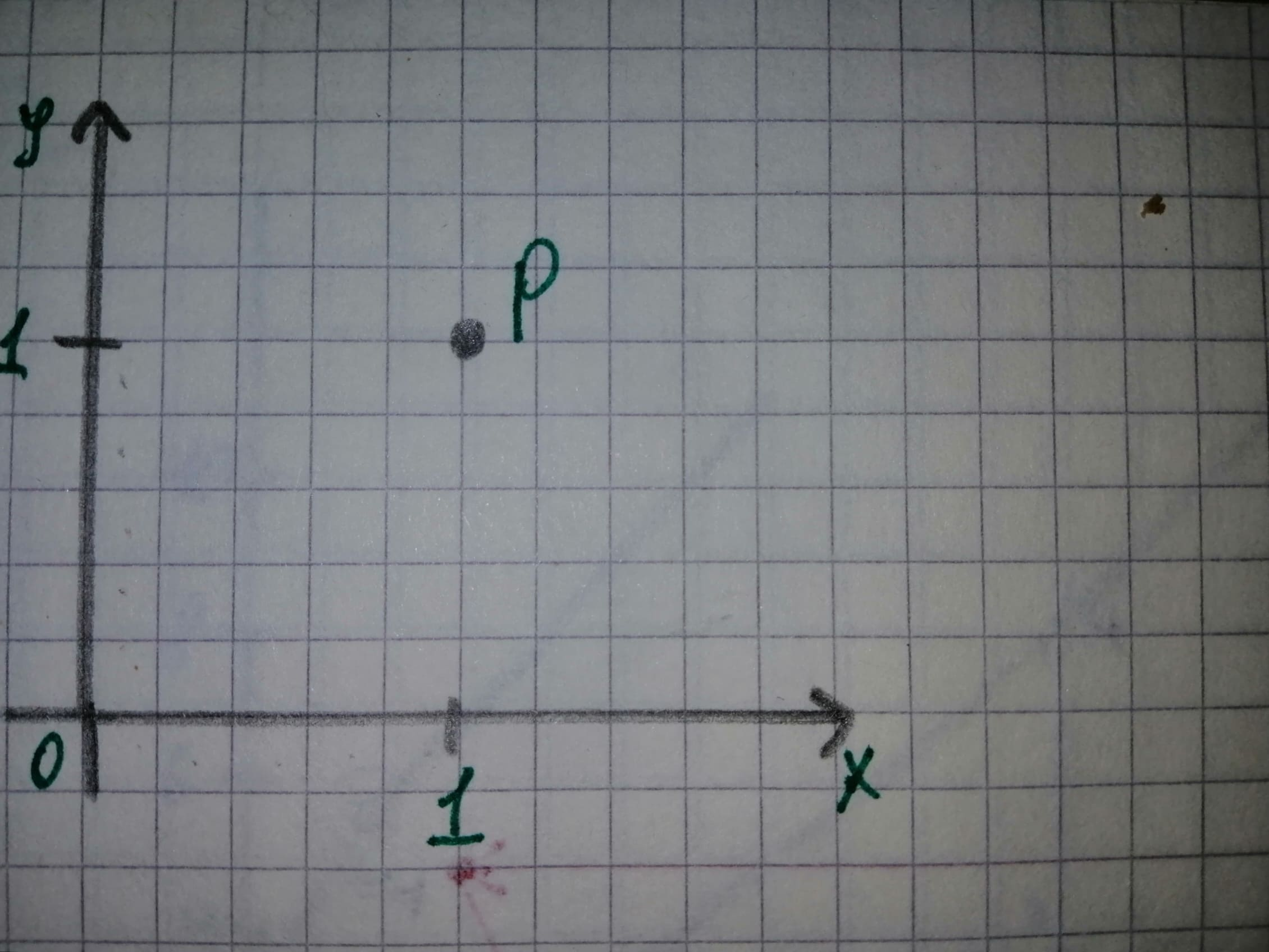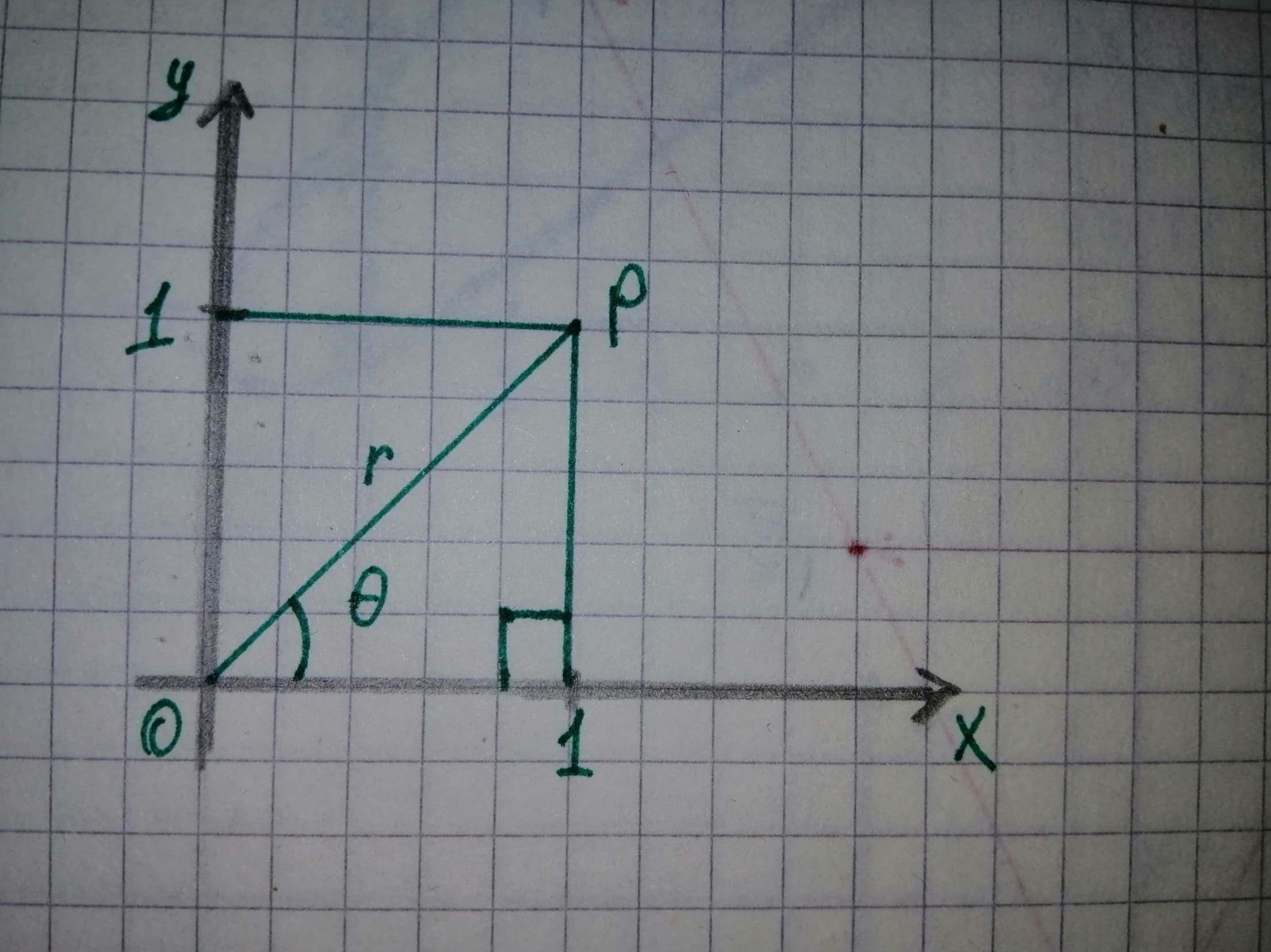usagirl007A

2020-12-29

The systems used to find the location of the point in a plane and find the rectangular and polar coordinates for the point P.Anonym

Given:
The point P is given in the figure belowThe below figure shows the relationship between the rectangular coordinates, (x, y) and polar coordinates $\left(r,\theta \right).$The Figure (1) shows that the point P has rectangular coordinates(1, 1).
Therefore, the value of x is 1 and the value of y is 1.
Formula to find r
$r=\sqrt{{x}^{2}+{y}^{2}}.$
Substitute 1 for x and 1 for y in above formula.
$r=\sqrt{{1}^{2}+{1}^{2}}$
$=\sqrt{2}$
Formula to find $\theta$
$\mathrm{tan}\theta =\frac{y}{x}$
Substitute 1 for x and 1 for y in above formula.
$\mathrm{tan}\theta =\frac{1}{1}$
$\theta ={\mathrm{tan}}^{-1}\left(1\right)$
$=\frac{\pi }{4}$
$\left({\mathrm{tan}}^{-1}\left(1\right)=\frac{\pi }{4}\right)$
Therefore the value of r is $\sqrt{2}$ and value of $\theta is\frac{\pi }{4}.$
Thus the rectangular coordinates for point P are (1, 1) and coordinates are $\left(\sqrt{2},\frac{\pi }{4}\right).$

Do you have a similar question?